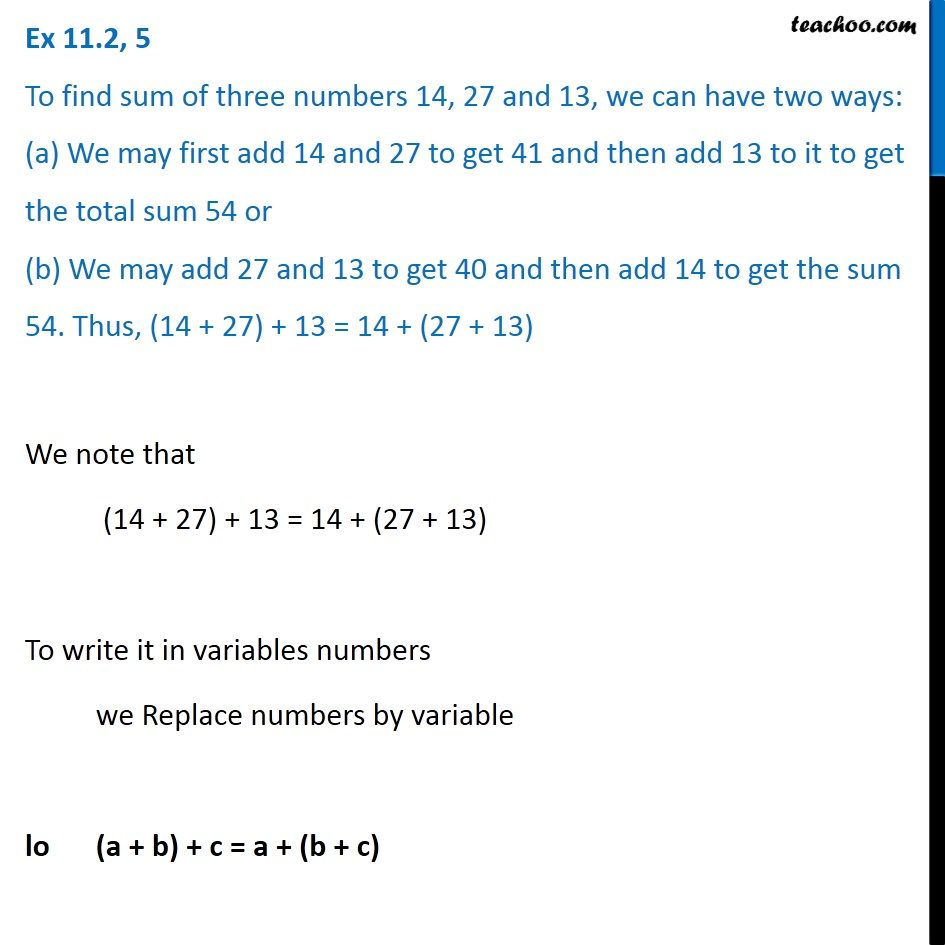Ex 11.2

Chapter 11 Class 6 Algebra
Serial order wiseGet live Maths 1-on-1 Classs - Class 6 to 12

### Transcript

Ex 11.2, 5 To find sum of three numbers 14, 27 and 13, we can have two ways: (a) We may first add 14 and 27 to get 41 and then add 13 to it to get the total sum 54 or (b) We may add 27 and 13 to get 40 and then add 14 to get the sum 54. Thus, (14 + 27) + 13 = 14 + (27 + 13) We note that (14 + 27) + 13 = 14 + (27 + 13) To write it in variables numbers we Replace numbers by variable lo (a + b) + c = a + (b + c)